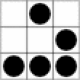/ logic

# Logic Symbols

## Logic symbols from Deductive Logic by Terry Halpin

hook
implies if ... then
p ⊃ q is false iff p is true and q is false
pqp ⊃ q
111
100
011
001
& ampersand
p & is true iff both p and q are true
pqp & q
111
100
010
000
wedge
p ∨ is true iff at least one of p, q are true
pqp ∨ q
111
101
011
000
tribar
p ≡ is true iff p and q have the same truth values
pqp ≡ q
111
100
010
001
!≡ slashed tribar*
p !≡ is true iff p and q have opposite truth values
pqp !≡ q
110
101
011
000
*no html entity equivalent
~ tilda
not p
p~p
10
01
Converse
The converse of the conditional is found by swapping the antecedent and the consequent
Original: If John has ten dollars then John has some money.
Converse: If John has some money then John has ten dollars.
p is contradictory to q iff p has the opposite truth value to q in all possible worlds
pqp & q~p ∨ ~ q
1110
1001
0101
0001

Content found within Wikipedia and the out of print book Deductive Logic by Terry Halpin